# Optimizing an indoor routing A*

Back in 2008 I was working at NAVTEQ, who had just been bought by Nokia, in the Madrid's R&D offices. There, the coolest project I worked at by far was optimizing an internal tool used to create indoor routing paths from grayscale images of interior maps.

It had lots of cool features, but I focused on trying to make its A * search algorithm and heuristics as fast as possible, to try to evolve the application into something usable in realtime, for example as a web service, and scalable to theorically hundreds of concurrent users.

It was going to be field tested at venues, so I went for hardcore scenarios like labyrinths instead of boring (and easy) corridors and square rooms.

This is a small summary of how it worked and how did I optimized it, based on the notes I took back then and the slides I prepared when I presented the project. Plus remembering as much as I could about A*, which I haven't touched again in +5 years. So bear with me if I make some mistake here or there...

1) Create the map mesh

Differentiate between walls and floor, or walkable/non-walkable tiles.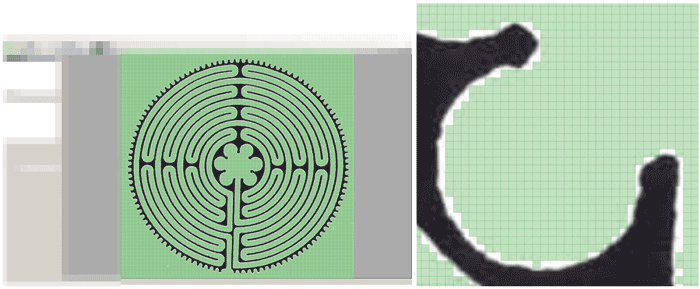The mesh resolution is quite important to avoid inaccuracies: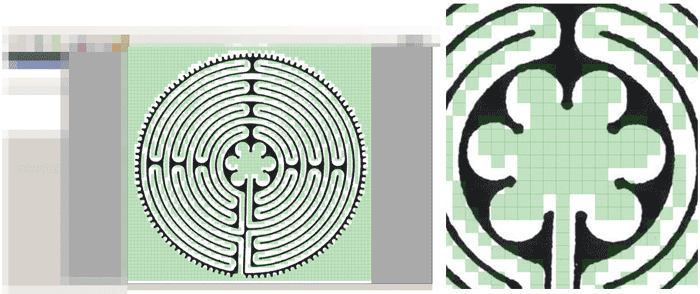Which can lead even to errors by "walking through walls" if the resolution is too small: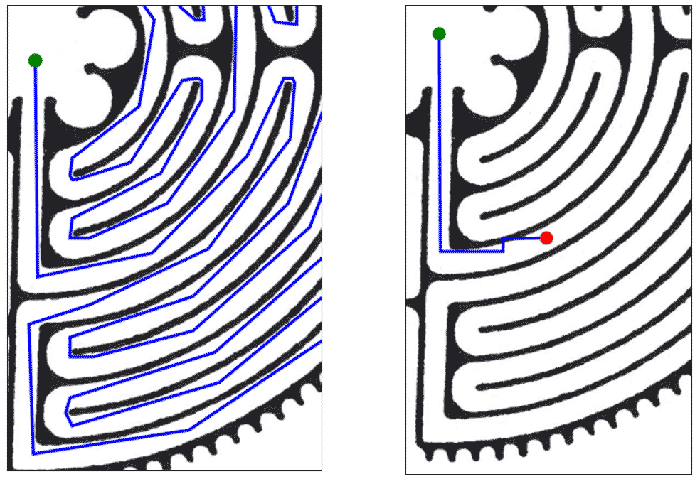2) Calculate costs mesh

Assign each walkable tile a cost value. Based on its distance from the walls, the further, the higher the cost, because the algorithm wants to travel as less tiles/spaces as possible.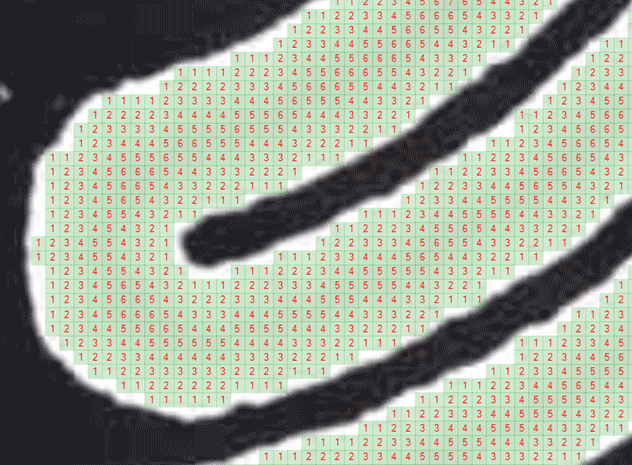3) Run the A * search

Then we can finally run the A * and let it map a route. Note that line simplifications and edge/turn detections are performed to obtain a more realistic navigation line drawing.

We'll get into what those yellow points mean right now. Let's just say that the default A * algorithm used them sometimes as "desirable positions" but as they cost a lot, if the heuristics found a faster route would tend to ignore them. The tool had implemented 3 different heuristics for short-circuiting the A * with a good enough solution, results would vary depending on which was used.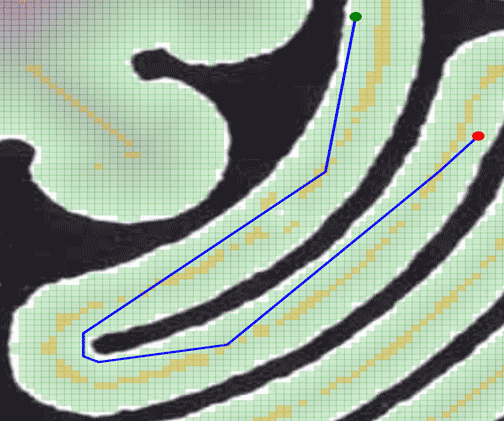This approach works and gets nice results, but it consumes a lot of CPU and needs to keep thousands of points in the mesh. Even counting with storing a pre-calculated points mesh and cost mesh for each map, it wasn't fast enough.

My Optimizations

1) Mark cost mesh maximas (already done)

Both due to A * trying to find the shortest path and due to our previously calculated cost mesh, if we run the A * right now, it would calculate the shortest route... but walking as near to the wall as possible.

Neither human beings usually walk touching the wall nor we want visually to look "so optimized", so instead, we will mark for the A * only the local maximas, those points inside a given radius that cost most (because are placed in the middle of corridors, rooms, etcetera and are less optimal for fastest paths).

This always has a maximum radius of calculation, so in huge rooms if the radius is not enough you might miss some points.

Maximas were already calculated to work as "attractors" for the algorithm when searching for the path, to try sometimes to make the line stick to the middle instead of going near the wall, but nothing else.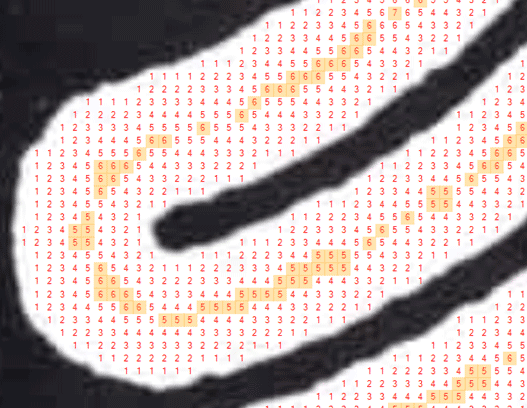2) Simplify the map to only use maximas

Go from almost 200k nodes...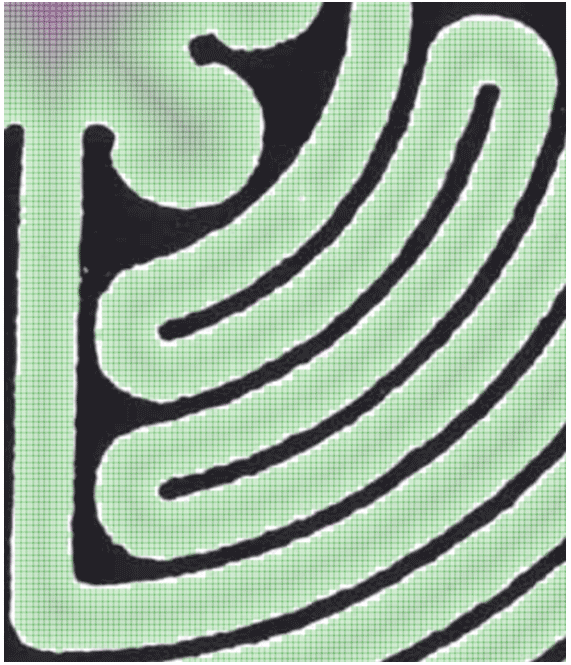To just ~300 keeping only the maximas, before any further optimization: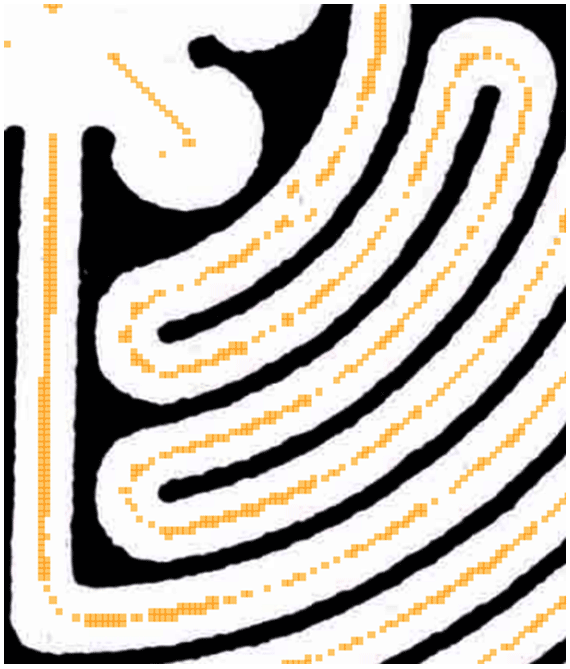3) Simplify the maximas and use them as navigation nodes

We can apply a line simplification algorithm and edge/turn detections (if angle is greater than x degrees from current direction vector, consider it a turn/edge and do not remove that point) to get rid of many maximas.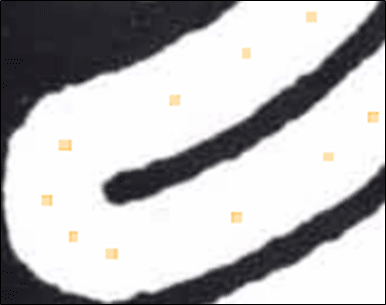And if we then apply a line of sight optimization... we can reduce even more!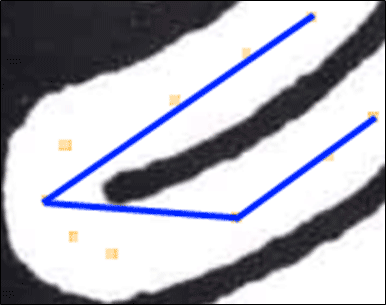Storing just 4 nodes for two corridors and a 180 degree turn is really nice (points used are those on edges of blue lines).

As the resulting line would be a bit ugly for a GPS-like representation, we could still store the pre-LOS nodes list to smooth the line into splines if we wanted to do so. After all what matters here is to calculate the A *, not the actual drawing of the line, which would be done client-side.

Having so few points was quite trivial to apply a Diagonal Distance heuristic and have a really fast A * applied in realtime.

Extra) Cache the costs

This might sound trivial, but whenever you can, cache the cost mesh, calculate it once and do not recalculate until needed. And then, try to only recalculate affected areas.

This is useful for example in a tower defense game, where you only need to recalculate enemies path whenever you place a new "soldier", or an RPG where you are moving inside a map that it's fixed and only needs to calculate a new path when the player clicks in a new position or object.

Even before the optimizations, having binary serialized meshes with pre-calculations instead of on-demand map processing saved seconds of initial load.

Conclusions

I don't remember the exact numbers I benchmarked, but I recall that the original prototype required Gigabytes of RAM to process any non-trivial black and white map, while the final prototype increased RAM usage upon loading the same image by less than 100MB, and we went from long calculations taking more than half a minute, to sub-second results (which I'm pretty sure could be further improved).

Thinking about this optimizations and actually making the application perform them (including tests!) has been of the most interesting projects I've done.

I don't know if this, along with other projects, were used in some indoor mapping Nokia software, but there were some other really cool ideas and PoCs when our Madrid R&D office was shut down.

Optimizing an indoor routing A* published @ . Author: